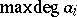# Effective Nullstellensatz

Let $f , f _ { 1 } , \dots , f _ { m } \in R : = k [ x _ { 1 } , \dots , x _ { n } ]$, where $k$ is a field. Hilbert's Nullstellensatz [a5] says that if $f$ vanishes on all the common zeros of the $f_i$ with coordinates in an algebraic closure of $k$, then, for some integer $\rho$, $f ^ { \rho } \in I : = ( f _ { 1 } , \dots , f _ { m} )$, i.e. there exist $a _ { 1 } , \dots , a _ { m } \in R$ such that

\begin{equation*} f ^ { \rho } = a _ { 1 } f _ { 1 } + \ldots + a _ { m } f _ { m }. \end{equation*}

An effective Nullstellensatz gives information on some aspect of the complexity of such a representation.

## Degree bounds.

If $\operatorname { deg } f _ { i } \leq d$, how large might one have to take $\rho$ and?

The projective version of the Masser–Philippon/Lazard–Mora example shows that this bound must be at least $d ^ { n }$. Although classical work of G. Hermann [a8] produces an explicit bound [a14], it is known that $d ^ { n }$ is the correct one [a16], [a17], [a11].

## Height bounds.

How large might a common denominator or the numerators of the $a_i$ be?

Assume, in addition, that $k = \mathbf{Q}$ and that when the coefficients of all the $f_i$ are put over a common denominator, the absolute values of that denominator and the numerators of the coefficients are at most $H > 0$. One can, of course, first bound the degrees, and then apply estimates from linear algebra to obtain a bound of the unfortunate shape $( d H ) ^ { c _ { n } d ^ { n^{2} } }$. In fact, by [a15], a denominator of absolute value at most $\operatorname{exp} c _ { n } d ^ { n } ( d + h ) q$ suffices, with explicit $c _ { n }$. The same is true of the numerators if one allows slightly larger than optimal bounds on the degrees of the $f_i$: $\operatorname { deg } f _ { i } \leq c _ { n } d ^ { n }$ [a2], [a3], [a13].

## Complexity bounds.

In the various models of computation, how many steps might be involved in finding such a representation?

In these models, as in the above classical case for heights, the complexity bounds turn out to be better than that obtained by simply applying linear algebra and using the degree bounds. Many interesting aspects, such as sparsity of the coefficients, arise. See [a9] for an introduction to a sizable and growing literature.

## Generalizations.

Various effective generalizations of Hilbert's Nullstellensatz exist, cf. [a19], [a6], [a12], as well as insight into important special situations [a10]. See [a1] for the link between the complexity of the Nullstellensatz and the problem $\mathcal{P} \neq \mathcal{N} \mathcal{P}$ (cf. $\cal N P$).

How to Cite This Entry:
Effective Nullstellensatz. Encyclopedia of Mathematics. URL: http://encyclopediaofmath.org/index.php?title=Effective_Nullstellensatz&oldid=50695
This article was adapted from an original article by W. Dale Brownawell (originator), which appeared in Encyclopedia of Mathematics - ISBN 1402006098. See original article